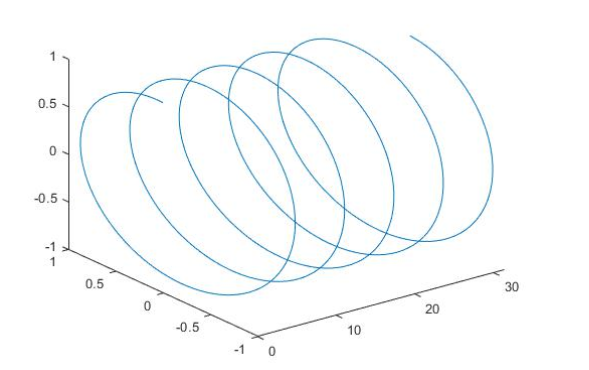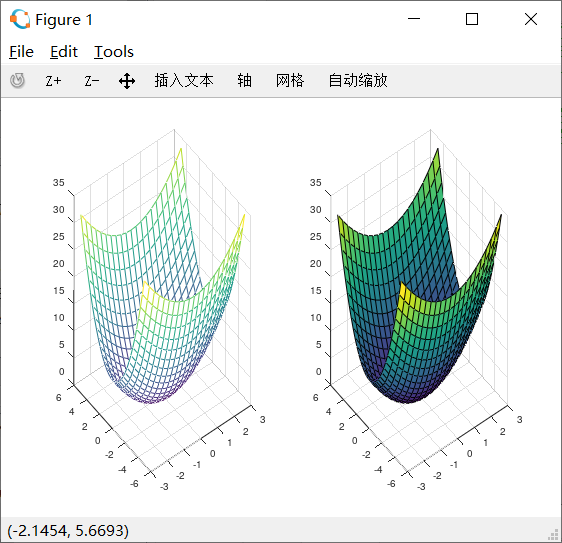plot3(X,Y,Z,s) % X、 Y、 Z 分别表示三维曲线的三轴坐标， s 为定义线型等信息的字符串

``````x = 0: pi/50: 10*pi;
y = sin(x);
z = cos(x);
plot3(x,y,z);
````````````[X,Y]=meshgrid(x,y) %将向量 x、 y 转换为网格数据矩阵 X、 Y
``````

``````mesh(X, Y, Z) % 绘制三维彩色网格曲面图
surf (X, Y, Z) % 绘制三维着色曲面图
``````

``````x = -3:0.3:3; y = -5:0.3:5; % 根据定义域按一定步长设定x、 y向量
[X,Y]=meshgrid(x,y); % 生成x-y平面的网格数据矩阵
Z = X.^2+ Y.^2; % 计算Z坐标， 注意用点运算
figure; % 新建一个窗口
subplot(1,2,1); mesh(X, Y, Z); % 在左子图绘制三维网格图
subplot(1,2,2); surf(X, Y, Z); % 在右子图绘制三维着色曲面图
``````